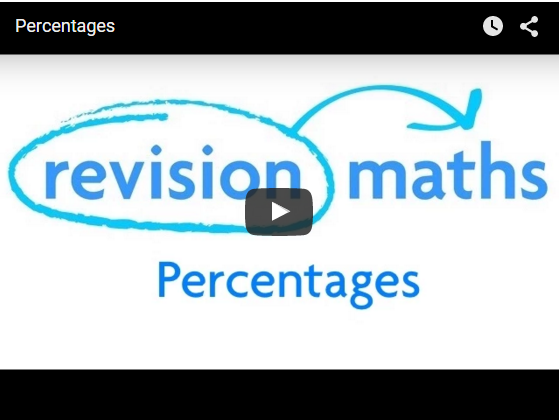## Percentages

Introduction

A percentage is a fraction whose denominator (bottom) is 100. So if we say 50%, we mean 50/100 = 1/2 (after cancelling). So 50% means ½. If want to find 10% of something, 'of' just means 'times'. So 10% of 150 = 10/100 × 150 = 15.

If you have to turn a percentage into a decimal, just divide by 100. For example, 25% = 25/100 = 0.25. To change a decimal into a percentage, multiply by 100. So 0.3 = 0.3 × 100 =30% .

Example

Find 25% of 10 (remember 'of' means 'times').

25/100 × 10

= 2.5

The video below shows you how to handle some exam questions regarding percentage, including: turning decimals into fractions, how to calculate the percentage of a value, calculating percentage change and calculating compound interest.Percentage Change

% change = new value - original value × 100

original value

Example

The price of some apples is increased from 48p to 67p. By how much percent has the price increased by?

% change = 67 - 48 × 100

48

= 39.58%

Percentage Error

% error = error × 100

real value

Example

Nicola measures the length of her textbook as 20cm. If the length is actually 17.6cm, what is the percentage error in Nicola's calculation?

% error = 20 - 17.6 × 100 = 13.64%

17.6

Original value

Original value = New value × 100

100 + %change

Example

Amish buys a stamp collection and makes a 35% profit by selling it for £2700. Find the cost of the collection. It is the original value we wish to find, so the above formula is used.

2700 × 100 = £2000

100 + 35

Percentage Increases and Interest

New value = 100 + percentage increase × original value

100

Example

£500 is put in a bank where there is 6% per annum interest. Work out the amount in the bank after 1 year.

In other words, the old value is £500 and it has been increased by 6%.

Therefore, new value = 106/100 × 500 = £530 .

Compound Interest

If in this example, the money was left in the bank for another year, the £530 would increase by 6%. The interest, therefore, will be higher than the previous year (6% of £530 is more than 6% of £500). Every year, if the money is left sitting in the bank account, the amount of interest paid would increase each year. This phenomenon is known as compound interest.

The simple way to work out compound interest is to multiply the money that was put in the bank by nm, where n is (100 + percentage increase)/100 and m is the number of years the money is in the bank for, i.e:

(100 + %change)no of years × original value

So if the £500 had been left in the bank for 9 years, the amount would have increased to:

500 × (1.06)9 = £845

Percentage decreases

New value = 100 - percentage decrease × original value

100

Example

At the end of 2003 there were 5000 members of a certain rare breed of animal remaining in the world. It is predicted that their number will decrease by 12% each year. How many will be left at the end of 2005?

At the end of 2004, there will be (100 - 12)/100 × 5000 = 4400

At the end of 2005, there will be 88/100 × 4400 = 3872

The compound interest formula above can also be used for percentage decreases. So after 4 years, the number of animals left would be:

5000 x [(100-12)/100]4 = 2998